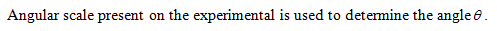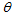# In the grating equation nλ=d sin θ, the quantity θ will be determined in the lab

In the grating equation nλ=d sin θ, the quantity θ will be determined in the lab.sticks to measure the distances and calculateusing geometry/trigonometry.